# Python If Statements in Field Calculator

6252
11
03-11-2016 07:00 AM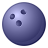Occasional Contributor

Hello all,

I am trying to convert a nested IF statement from Excel into Python via the Field Calculator in ArcMap. The data is wind data which has two fields: U and V; and the calculation is to be entered in the DIR field. These are vectors can be applied with triganometry to create wind direction in degrees. In Excel, the DIR field has the following formula:

=IF(D2>0,((180/PI())*ATAN(C2/D2)+180),IF(AND(C2<0,D2<0),((180/PI())*ATAN(C2/D2)),IF(AND(C2>0,D2<0),((180/PI())*ATAN(C2/D2)+360),IF(AND(D2=0,C2>0),270,IF(AND(D2=0,C2<0),90,0)))))

...where column C is the U field and column D is the V field.

I've been playing all day with this and so far, I have the following:

codeblock:

```def winddir(DIR):
if !V! > 0:
!DIR! = ((180/math.pi)*math.atan(!U!/!V!)+180)
elif !U! < 0 and !V! < 0:
!DIR! = ((180/math.pi)*math.atan(!U!/!V!))
elif !U! > 0 and V < 0:
!DIR! = ((180/math.pi)*math.atan(!U!/!V!)+360)
elif !V! == 0 and !U! > 0:
!DIR! = 270
elif !V! == 0 and !U! < 0:
!DIR! = 90```

DIR =

`winddir(DIR)`

I keep getting the generic error:

ERROR 000989: Python syntax error: Parsing error SyntaxError: invalid syntax (line 2)

I'm really pulling my hair out with this as I've tried tweaking the syntax but I cannot resolve it. Any assistance would be greatly appreciated.

Thanks,

DK

Tags (5)
11 Replies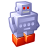Regular Contributor II
```import math
def winddir(DIR, V, U):
if V > 0:
return (180/math.pi) * math.atan(U/V) + 180
elif U < 0 and V < 0:
return (180/math.pi) * math.atan(U/V)
elif U > 0 and V < 0:
return (180/math.pi) * math.atan(U/V) + 360
elif V == 0 and U > 0:
return 270
elif V == 0 and U < 0:
return 90

winddir(!DIR!, !V!, !U!)```

Calculate Field examples—Help | ArcGIS for DesktopNew Contributor III
```import math
k = (180/math.pi)

def winddir(v,u):
v = float(v)
u = float(u)
if v > 0:
result = (180/math.pi)*math.atan(u/v)+180)
elif u < 0 and v < 0:
result = ((180/math.pi)*math.atan(u/v))
elif u > 0 and v < 0:
result = ((180/math.pi)*math.atan(u/v)+360)
elif v == 0 and u > 0:
result = 270
elif v == 0 and u < 0:
result = 90
else: result = -1
return result

#-------------------
windir(!V!,!U!)```

Line 21 belongs to the expression-line, L1- 18  is part of the codeblock

So the expression in L21 calls the windir function (L4-18) for every feature in the table with the current values of the fields V and U.

Dir is the targetfield for the result of the function. For the function itself it's not nessesary.

Line 1&2 will be evaluated only one time at the start of calculation.

The following lines will be evaluated as a part of the windir-function every feature/record

L4: The function to calculate the wind direction needs only two parameters: The values of the fields U and V.

L5&6: You have to make shure, that the variables have the correct datatype.

L8,10,12,14&17: The variable is defined by the calculation and at ...

L18 at least by the word return the function has the instruction to  "give the value back to the one who calls you up"

It's more "pythonical" to do it like Freddie, but for beginners/examples  my contruction is easyer to understand.

L17: Every time you  use an if statemant, IMO you have to use else at the end to capture all other oportunities.New Contributor III

Uups, because you calculate only with integer it's better to use int() then float().Occasional Contributor

Thanks to both for the feedback. Can I ask why the fields are only referenced in the expression line and not in the codeblock? I'm currently teaching myself Python so I haven't covered all the theory yet!

Also, as I have ~50 shapefiles to apply this code to, I've run Freddie's code in Field Calculator which worked well (I've added some extra elif's at the end to cover all possibilities. However, when I copy the successful code as a Python snippet from the Results window, amend the shapefile name and run again, I get a similar syntax issue as before. The code I'm trying to run from the Python snippet is below:

`arcpy.CalculateField_management("New Group Layer/09-03-2016 02_00_00","DIR","winddir(!DIR!, !V!, !U!)","PYTHON_9.3","import math  /ndef winddir(DIR, V, U):  /n    if V > 0:  /n        return (180/math.pi) * math.atan(U/V) + 180  /n    elif U < 0 and V < 0:  /n        return (180/math.pi) * math.atan(U/V)  /n    elif U > 0 and V < 0:  /n        return (180/math.pi) * math.atan(U/V) + 360  /n    elif V == 0 and U > 0:  /n        return 270  /n    elif V == 0 and U < 0:  /n        return 90/n    elif U == 0 and V > 0:  /n        return 180  /n    elif U == 0 and V < 0:  /n        return 0 /n    elif U == 0 and V == 0:  /n        return 0")`

I've only changed one digit in the file name from the Python snippet (01_00_00 to 02_00_00) in the above code and I'm getting the following error:

Runtime error  Traceback (most recent call last):   File "<string>", line 1, in <module>   File "c:\program files (x86)\arcgis\desktop10.2\arcpy\arcpy\management.py", line 3354, in CalculateField     raise e ExecuteError: ERROR 000989: Python syntax error: Parsing error SyntaxError: invalid syntax (line 1)

DKbyMVP Esteemed Contributor
 Thanks to both for the feedback. Can I ask why the fields are only referenced in the expression line and not in the codeblock? I'm currently teaching myself Python so I haven't covered all the theory yet!

The reason for this is that the Calculate Field expression is interpreted row by row as you go through the table - the field expression !FIELDNAME! are converted to a value, say, 1024.4, and then that expression is executed.   A similar thing is done with the VBScript parser with a different syntax [FIELDNAME]. (I recommend sticking with the Python parser.)

The code in the function is not involved in this field name to value substitution process which is why you use real variables in the code block (passed to the function as arguments).

There are nice examples in the help that make more sense if you know this. There's a help article on Calculate Field examples that I refer to again and again! I recommend bookmarking it!

Calculate Field examples—Help | ArcGIS for Desktop

Calculate Field is much easier to use in ModelBuilder and from tool dialogs.  Cursors are often a better approach in scripting and at the python command line.Esteemed Contributor

As the “VB” is no longer available within the “field calculator” tool, I couldn’t figure out how to write a code for the if statement in Python for the 5 cases shown in the screenshot below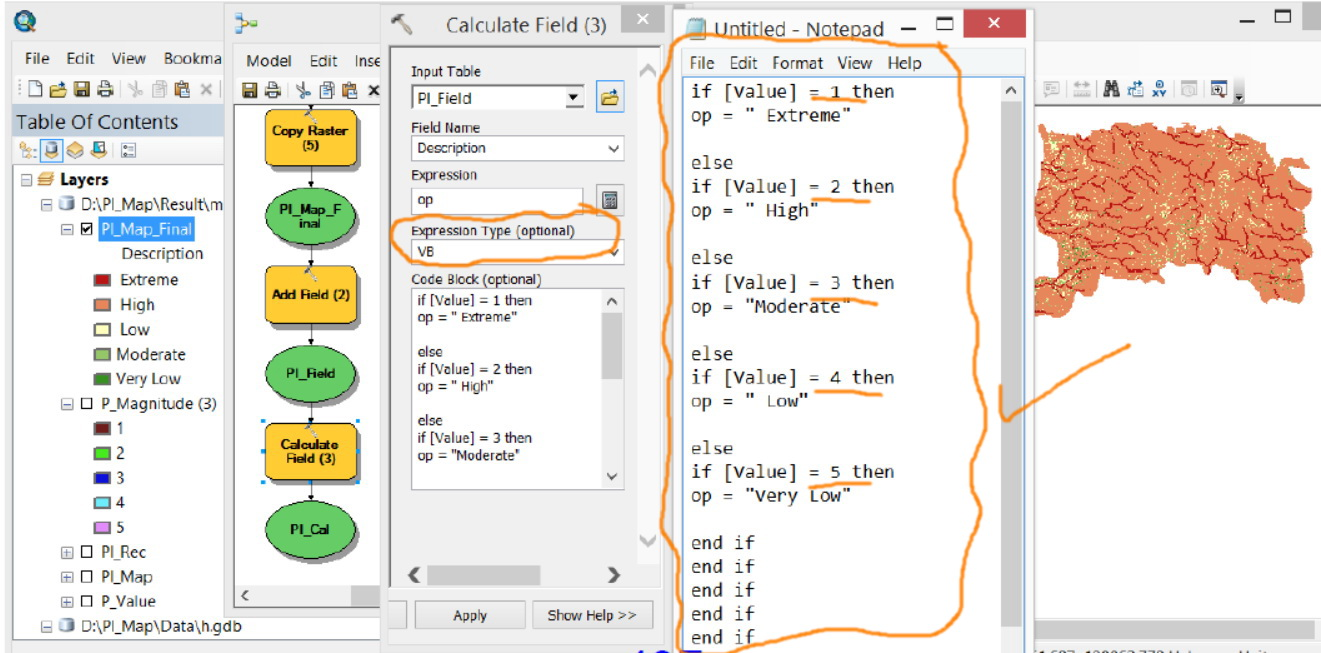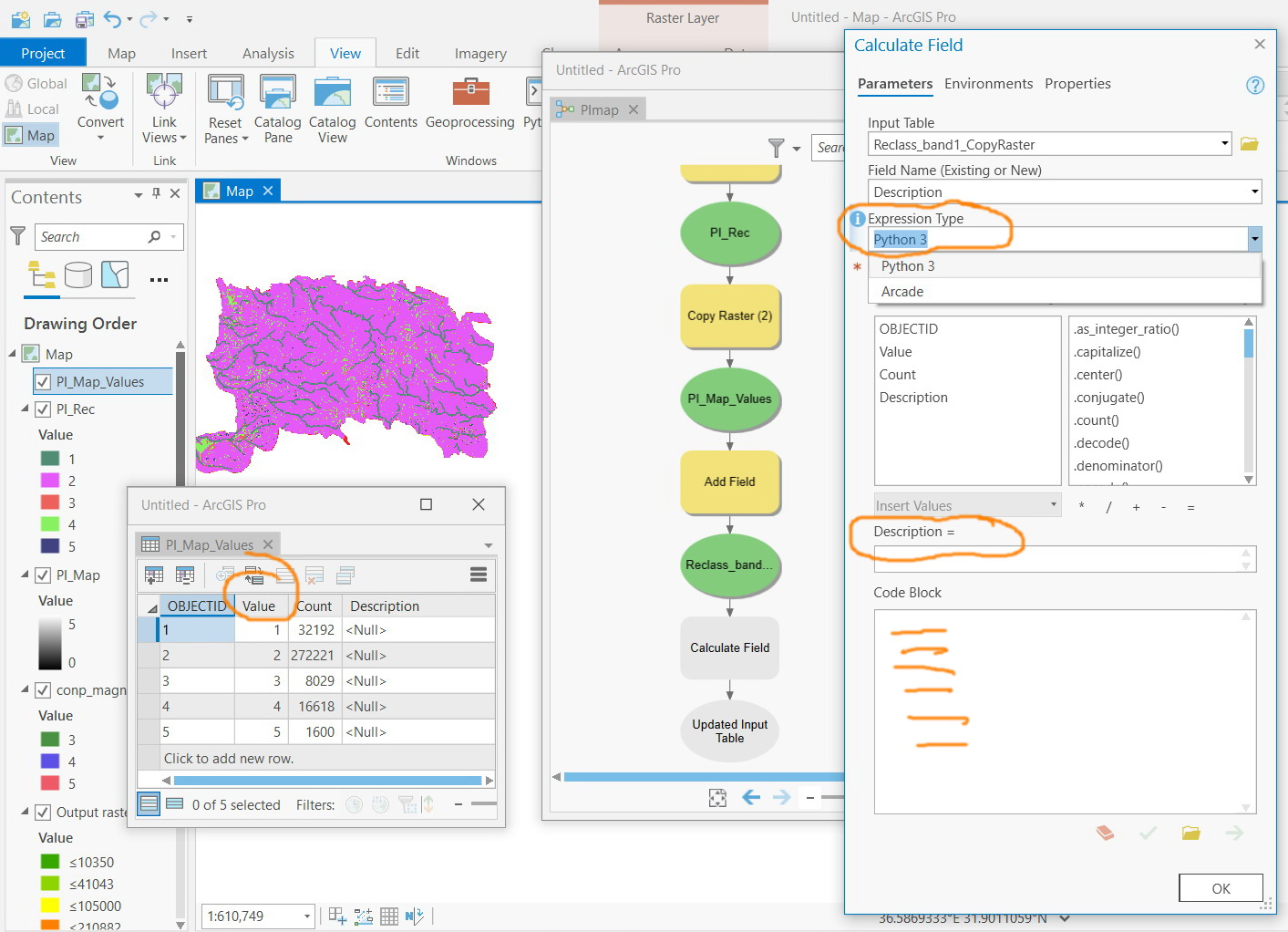----------------------------------------
Jamal Numan
Geomolg Geoportal for Spatial Information
Ramallah, West Bank, PalestinebyMVP Esteemed Contributor

Hi Jamal,

If they are that sequential (1-5) you can do this one line Python expression:

``["Extreme", "High", "Moderate", "Low", "Very Low"][!FIELD!]‍ - 1]  # corrected‍``Esteemed Contributor

Many thanks Curtis for the help.

The code ends up with an error. What could be the issue here?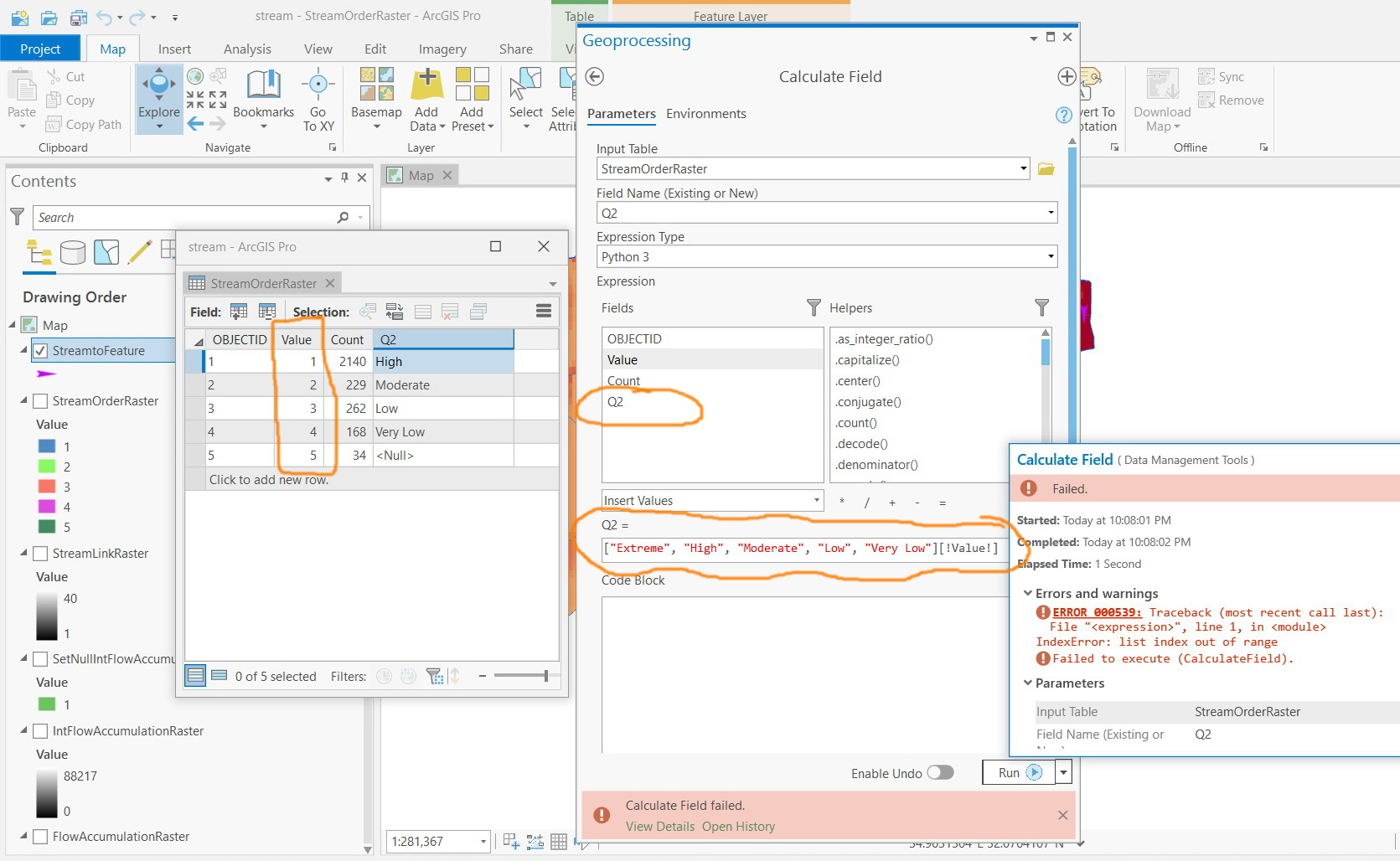----------------------------------------
Jamal Numan
Geomolg Geoportal for Spatial Information
Ramallah, West Bank, PalestinebyMVP Esteemed Contributor

OOPS in Python it's zero based indexes  ([1,2,3] == 1) so use [!Value! - 1]

I will edit my post to be correct.